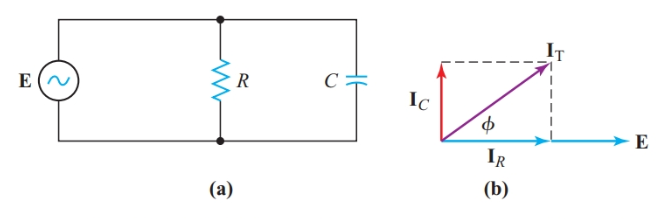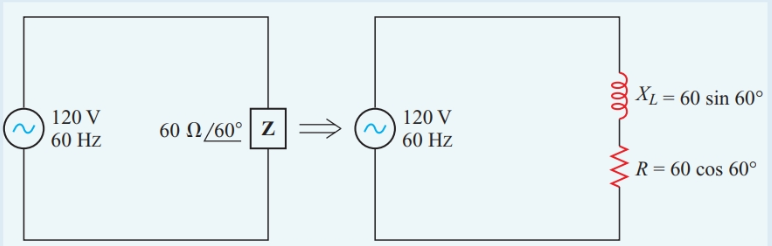Home / Electric Power / Power Triangle and Power Factor in AC Circuits

# Power Triangle and Power Factor in AC Circuits

Want create site? Find Free WordPress Themes and plugins.

## Power Triangle

The real power in the circuit of Figure 1 can be found from the product of VR and I, and the reactive power from the product of VL and I. Since VL leads VR by 90°,

$\begin{matrix}{{V}_{T}}=\sqrt{V_{R}^{2}+V_{L}^{2}} & {} & \left( 1 \right) \\\end{matrix}$Figure 1 AC circuit with resistance and inductance in series

Multiplying each side of Equation 1 by the common current gives

${{V}_{T}}I=\sqrt{{{\left( {{V}_{R}}I \right)}^{2}}+{{\left( {{V}_{L}}I \right)}^{2}}}$

Therefore,

$\begin{matrix}S=\sqrt{{{P}^{2}}+{{Q}^{2}}} & {} & \left( 2 \right) \\\end{matrix}$

Similarly, in the parallel circuit of Figure 2, the current in the capacitor branch leads the current in the resistor branch by exactly 90°. For this circuit, Current equation can be written as:

${{I}_{T}}=\sqrt{I_{R}^{2}+I_{C}^{2}}$

Multiplying each side by the common voltage gives

$V{{I}_{T}}=\sqrt{{{\left( V{{I}_{R}} \right)}^{2}}+{{\left( V{{I}_{C}} \right)}^{2}}}$

Therefore,

$S=\sqrt{{{P}^{2}}+{{Q}^{2}}}$Figure 2 AC circuit with resistance and capacitance in parallel

Note that Equation 2 applies to both series and parallel circuits. Since power is the product of current (the common phasor of series circuits) and voltage (the common phasor of parallel circuits), the same equations for power apply to series and parallel circuits as well as to series-parallel circuits.

Although instantaneous power varies sinusoidally, it does so at twice the frequency of the voltage and current. Consequently, we cannot draw power phasors on the same phasor diagram with voltage and current. Therefore, it is customary to represent the Pythagorean relationship of Equation 2 with right triangles, as shown in Figure 3.

In a power triangle, the horizontal side represents real power. The vertical side represents reactive power and forms a right angle at the right-hand end of the side representing real power. Since Equation 2 applies to either a series or a parallel circuit, we can develop a power triangle from either an impedance diagram or an admittance diagram.

If we start with an impedance diagram, in which inductive reactance is drawn in the +j direction, the power triangle appears as in Figure 3(a). But if we start from an admittance diagram, in which inductive susceptance is drawn in the -j direction, the power triangle has the form shown in Figure 3(b).

To avoid confusion between inductive and capacitive reactive power in power triangle diagrams, we must choose one of these formats for all AC circuits. We will follow the modern North American convention of showing inductive reactive power in the +j direction. With this convention, the source current is the reference phasor when we are dealing with power in AC circuits.Figure 3 Power triangle with (a) common current as a reference axis and (b) voltage as a reference axis

We can now determine the phase angle, ϕ, of the apparent power. With the power triangle drawn as shown in Figure 3(a), S has the same angle as the impedance of the circuit and as the conjugate of the admittance of the circuit (in other words, the angle of the admittance is -ϕ). When S is treated as a complex number, it is called the phasor power of the circuit. Thus,

$S=P+jQ$

We can now see where the terms real power for active power and quadrature (or imaginary) power for reactive power originated. Since inductive reactive power has a +j operator, capacitive reactive power has a -j operator.

Since the voltage across the capacitance in a series circuit is 180° out of phase with the voltage across the inductance, the net reactive voltage is the difference between the two reactive voltages.

Similarly, in a simple parallel circuit, the net reactive current is the difference between the capacitive and inductive branch currents. Since Q = VXIX, the net reactive power in both series and parallel AC circuits is the difference between the inductive reactive power and the capacitive reactive power.

In Figure 4 the instantaneous power in inductance is positive when the current is rising and building up a magnetic field around the inductor. But Figure 5 shows that, when the current is rising, the capacitance is discharging its stored energy back into the system. Therefore, in an AC circuit containing both inductance and capacitance, the capacitance always returns energy to the circuit when the inductance takes energy from the circuit, and vice versa.

Consequently, some energy is transferred back and forth between the inductance and the capacitance of the circuit, and the net reactive power to and from the source is the difference between the inductive reactive power and the capacitive reactive power.Figure 4 Instantaneous power in an ideal inductorFigure 5 Instantaneous power in a capacitor

Example 1

A circuit has two branches connected in parallel to a 120-V 60-Hz source. Branch 1 consists of a 75-Ω resistance and a 100-Ω inductive reactance in series, and branch 2 consists of a 200-Ω capacitive reactance. Determine the apparent power of the circuit.

Solution

Step 1

Draw a circuit diagram as shown in Figure 6. Then calculate the current in branch 1:

\begin{align}& {{Z}_{1}}=R+J{{X}_{L}}=75+j100=125\angle +{{53.1}^{o}}\Omega \\& {{I}_{1}}=\frac{E}{{{Z}_{1}}}=\frac{120V}{125\Omega }=0.960A \\\end{align}Figure 6 Diagrams for Example 1

Step 2

Draw a power triangle and calculate the net reactive power. The real power of the circuit is

$P=I_{1}^{2}R={{0.960}^{2}}\times 75=69.1W$

Reactive power of the inductance is

$Q=I_{1}^{2}{{X}_{L}}={{0.960}^{2}}\times 100=92.2W$

Reactive power of the capacitance is

$Q=\frac{{{E}^{2}}}{{{X}_{C}}}=\frac{{{120}^{2}}}{200}=72VAR$

Net reactive power of the circuit is

$Q=92.2-72=20.2VAR\left( Inductive \right)$

Step 3

Calculate the apparent power:

$S=\sqrt{{{P}^{2}}+{{Q}^{2}}}=\sqrt{{{69.1}^{2}}+{{20.2}^{2}}}=72VA$

## Power Factor

Since the hypotenuse of a right triangle must be longer than either of the other two sides, the apparent power that a generator must supply to a reactive load is always greater than the real power that the load can convert into some other form of energy. This relationship can be quite important since most industrial loads have appreciable inductive reactance.

Power factor is the ratio between the real power and the apparent power of a load in an ac circuit.

The power triangle of Figure 3(a) shows that the ratio of real power to apparent power is the cosine of the power-factor angle between real power and apparent power.

Tracing the construction of the power triangle back through the impedance diagram to the phasor diagram for current and voltage, we find that the power-factor angle is the same as the phase angle, ϕ, between the voltage across and the current through the load.

From the definition of power factor,

$\begin{matrix}Power-Factor=\frac{P}{S}=\cos \phi & {} & \left( 3 \right) \\\end{matrix}$

We distinguish between inductive and capacitive loads by stating that inductive loads always have a lagging power factor because their currents lag behind their voltages, and that capacitive loads always have a leading power factor because their currents lead their voltages.

Since the real power cannot be greater than the apparent power, the power factor cannot be greater than 1. It can be expressed as either a decimal fraction or a percentage.

Example 2

Find the real power and power factor of a load whose impedance is 60 V∠+60° when connected to a 120-V 60-Hz source.

Solution

Step 1

Convert the impedance to rectangular coordinates to find an equivalent circuit consisting of resistance and reactance in series, as shown in Figure 7.

$Z=60\cos {{60}^{o}}+j60\sin {{60}^{o}}$

Therefore,

$R=60\cos {{60}^{o}}=60\times 0.500=60\Omega$Figure 7 Schematic diagram for Example 2

Step 2

\begin{align}& I=\frac{E}{Z}=\frac{120V}{60\Omega }=2A \\& P={{I}^{2}}R=4\times 30=120W \\\end{align}

Step 3

\begin{align}& S={{I}^{2}}Z=4\times 60=240VA \\& \cos \phi =\frac{P}{S}=\frac{120}{240}=0.5Lagging \\\end{align}

We can express real power in terms of power factor and apparent power. Since S = EI, the real power in an AC circuit is

$\begin{matrix}P=EI\cos \phi & {} & \left( 4 \right) \\\end{matrix}$

Once we know the real power and apparent power of an AC load, we can determine reactive power:

$\begin{matrix}Q=\sqrt{{{S}^{2}}-{{P}^{2}}} & {} & \left( 5 \right) \\\end{matrix}$

Substituting P = S cos ϕ gives

$\begin{matrix}Q=S\sqrt{1-{{\cos }^{2}}\phi } & {} & \left( 6 \right) \\\end{matrix}$

The ratio of reactive power to apparent power can be useful when we wish to solve directly for the reactive power of the load.

The reactive factor is the ratio of the reactive power to the apparent power of an AC load.

From the power triangle in Figure 3(a) we can see that

$\begin{matrix}Reactive-Factor=\frac{Q}{S}=\sin \phi & {} & \left( 7 \right) \\\end{matrix}$

Summary

• A power triangle shows the relationships among apparent power, real power, and reactive power in an AC circuit.
• The power factor of a load in an AC circuit is the ratio of real power to apparent power.
• The power factor is the cosine of the phase angle between the voltage across and the current through the load.
• The reactive factor is the ratio of reactive power to apparent power.
• The reactive factor is the sine of the phase angle between the voltage across and the current through the load.
Did you find apk for android? You can find new Free Android Games and apps.